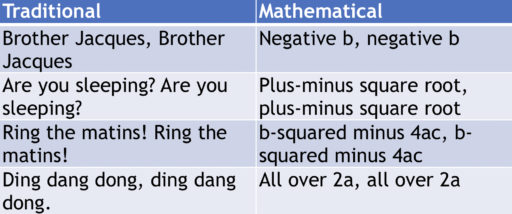In this post, we will discuss the most general way to solve a quadratic equation: the quadratic formula. If you only have enough time to learn one way of solving a quadratic equation, you should just memorize this formula (and skip this post on factoring) since this strategy is guaranteed to work (whereas sometimes factoring works well and sometimes not). Here is how the method works:

Suppose we have a quadratic equation of the form:Then, the solutions are:Note that (provided), this means that we will have 2 solutions for our equation: one where we add the square root and another where we subtract it. And in general, quadratic equations do have 2 solutions.

This is a very powerful formula and basically allows one to solve any quadratic equation, provided one is willing to learn the formula and, sometimes, do a little bit of manipulation to the initial equation.

Here is an example problem:

Example
Find the solution(s) to:This is a somewhat ugly equation so before proceeding, we do some multiplication to get rid of the fractions. Multiplying by 7, we get:And before using our formula, it is crucial that all the terms are on one side, and that the other side is equal to. Thus, we subtractto get:Now, we can apply the formula to get:Thus,.

Remembering the Formula
Using the quadratic formula is generally straightforward. The tricky part is remembering the formula itself. One way to do so is via a song. If you are familiar with the tune of “Brother Jacques,” you could sing the following:Practice Problems
Find the solutions to the following quadratic equations:

1.2.3.4.5.